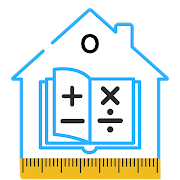# Construction Calculator All in One

## Easy way to calculate and convert construction quantities.

4.61 PRODUCTIVITY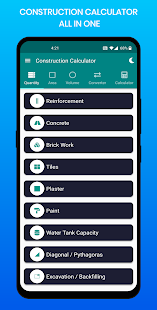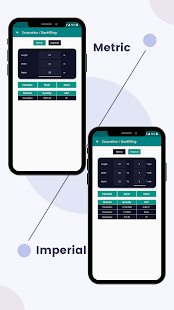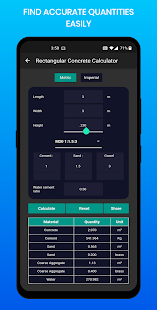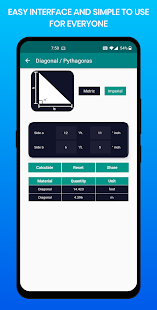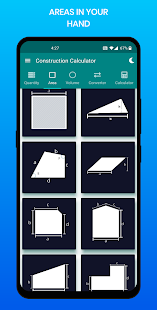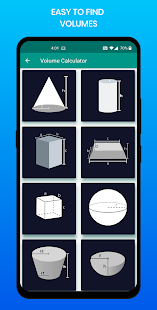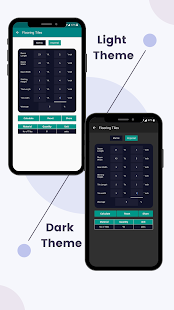Construction Calculator all in one can calculate with the Imperial Measurement System & Metric Measurement system. App also supports the Dark theme.
Construction Calculator All in one is a free android application for construction calculations. We use simple tools in the application to simplify the calculations for the construction industry. We help to calculate almost all kinds of area estimation calculation volume calculation unit converters and normal calculator as well.
We have divided the application into some parts like quantity calculator area calculator volume calculator unit converter and normal calculator.

This calculator is useful for Civil Engineer Site Supervisor Civil Engineering Student Mechanical Engineers Construction Project Managers Construction Store Manager Fresher Engineers Construction Contractors Building Contractors Store Keeper Site Execution engineers Estimation engineer and many more. Even a normal person who needs to do basic home calculations then he also needs this app. You can calculate the plot area so it will be useful for Real estate agents as well.
Quantity Estimation App For Construction
Quantity Calculation of Building Materials
Building calculator is divided into the following four categories-

Quantity Calculator includes-
-Reinforcement Steel Calculator
-Steel weight Calculator
-Concrete Calculator(with Volume without volume Circular Column)
-Excavation Calculator
-Backfill Calculator
-Brickwork Calculator
-Tile Calculator
-Plaster Calculator
-Paint Calculator
-Diagonal Calculator
-Pythagoras Calculator
-Water Tank Capacity Calculation(Circular and Rectangular)
-Water Tank Calculator
-Other Quantities and many more

Area Calculator includes-
-Area Measurement App
-Area Calculator for Land
-Circle Area Calculator
-Rectangle Area Calculator
-Triangle Area Calculator
-Rhombus Area Calculator
-L plot Area Calculator
-Square Area Calculator
-Right Angle Area Calculator
-Other areas and Many more

Volume Calculator includes-
-Sphere Volume Calculator
-Cube Volume Calculator
-Block Volume Calculator
-Bucket Volume Calculator
-Semi Sphere Volume Calculator
-Cone Volume Calculator
-Cylinder Volume Calculator
-Trapezoid Volume Calculator
-Rectangular Prism Volume Calculator
-Spherical Cap Volume Calculator
-Frustrum Volume Calculator
-Area Calculator Square Feet
-Other Volume Calculator and many more

Unit Convertor includes-
-Unit Converter For Civil Engineering
-Length Converter
-Weight Converter
-Area Converter
-Volume Converter
-Temperature Converter
-Pressure Converter
-Time Converter
-Speed Converter
-Fuel Converter
-Angle Converter
-Force Converter
-Power Converter
-Scientific Converter
-Unit Converter Calculator

This app features-
-Easy and simple to use
-Added Default standard values for Simplification
-Easy interface for everyone
-A non-technical person can use
-Gives accurate information
-Fast in Calculation
-Almost all construction calculations included
-Can reset data if an error in entry
-Normal Calculator is included

We appreciate all the feedback from your side. Your suggestions and advice will help us to improve our app. If you have any suggestions about the application then feel free to contact us by email binaryandbricks@gmail.com

### Video

*Added staircase in the concrete calculator *Added Imperial system in volume with no of units *Added dry volume in concrete calculator *No Of columns or slabs added to the concrete calculator *More practical concrete grades added *Minor bug fixes.

### Other versions

1.4.2 released on 06 October 2020 (101 days ago)

### Details and Recent Ratings

Size: 24 MB
Version: 1.5.0 by Binary and Bricks Pvt. Ltd.
Updated: 31 December 2020 (15 days ago)
Released: 10 August 2020
Installations: more than 50 000
5 Stars: 202
4 Stars: 22
3 Stars: 9
2 Stars: 4
1 Star: 9

### Similar apps

Android Game Categories
Android App Categories